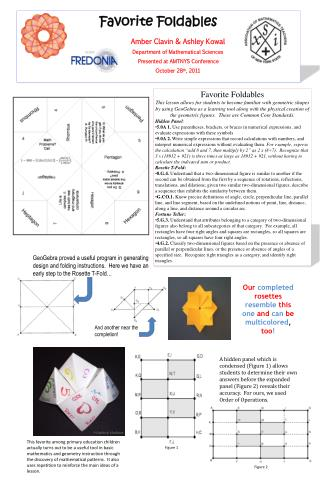DownloadDownload PresentationFavorite Foldables

# Favorite Foldables

Télécharger la présentation## Favorite Foldables

- - - - - - - - - - - - - - - - - - - - - - - - - - - E N D - - - - - - - - - - - - - - - - - - - - - - - - - - -
##### Presentation Transcript

1. Favorite Foldables Amber Clavin & Ashley Kowal Department of Mathematical Sciences Presented at AMTNYS Conference October 28th, 2011 • Favorite Foldables • This lesson allows for students to become familiar with geometric shapes by using GeoGebra as a learning tool along with the physical creation of the geometric figures. These are Common Core Standards. • Hidden Panel: • 5.0A 1. Use parentheses, brackets, or braces in numerical expressions, and evaluate expressions with these symbols • 5.0A 2. Write simple expressions that record calculations with numbers, and interpret numerical expressions without evaluating them. For example, express the calculation “add 8 and 7, then multiply by 2” as 2 x (8+7). Recognize that 3 x (18932 + 921) is three times as large as 18932 + 921, without having to calculate the indicated sum or product. • Rosette T-Fold: • 8.G.4. Understand that a two-dimensional figure is similar to another if the second can be obtained from the first by a sequence of rotations, reflections, translations, and dilations; given two similar two-dimensional figures, describe a sequence that exhibits the similarity between them. • G.CO.1. Know precise definitions of angle, circle, perpendicular line, parallel line, and line segment, based on the undefined notions of point, line, distance, along a line, and distance around a circular arc. • Fortune Teller: • 5.G.3. Understand that attributes belonging to a category of two-dimensional figures also belong to all subcategories of that category. For example, all rectangles have four right angles and squares are rectangles, so all squares are rectangles, so all squares have four right angles. • 4.G.2. Classify two-dimensional figures based on the presence or absence of parallel or perpendicular lines, or the presence or absence of angles of a specified size. Recognize right triangles as a category, and identify right triangles. GeoGebra proved a useful program in generating design and folding instructions. Here we have an early step to the Rosette T-Fold… Ourcompletedrosettesresemblethisoneandcanbemulticolored, too! And another near the completion! A hidden panel which is condensed (Figure 1) allows students to determine their own answers before the expanded panel (Figure 2) reveals their accuracy. For ours, we used Order of Operations. Students attempt to identify certain shapes used in Snowy Night. This favorite among primary education children actually turns out to be a useful tool in basic mathematics and geometry instruction through the discovery of mathematical patterns. It also uses repetition to reinforce the main ideas of a lesson. Figure 1 Figure 2